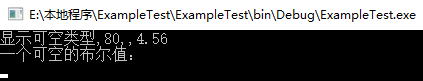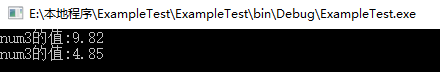# C#可空类型知多少

2019/04/10 10:10

##### 1、可空类型基础知识

T? myNullableNum = rangedNum/new T?() or null;


int? intClass = null;


int? intClass = new int?()


Nullable<int> myNullableClass = new Nullable<int>();


##### 2、可空类型的使用

int? k=10;

Nullable<int> k = new Nullable<int>(10);

int m;  //默认值为0
int? m; //默认值为null


int? num = null;
var k == num ?? 9;


<data_type>?<variable_name> = null;


using System;

namespace ExampleTest
{
class Program
{
static void Main(string[] args)
{
int? objectNull = null;
int? k = 80;
double? db1 = new double?();
double? db2 = 4.56;
bool? b = new bool?();

Console.WriteLine($"显示可空类型{objectNull},{k},{db1},{db2}"); Console.WriteLine($"一个可空的布尔值：{b}");
}
}
}using System;

namespace ExampleTest
{
class Program
{
static void Main(string[] args)
{
double? objNull = null;
double? k = 4.85;
double db;
db = objNull ?? 9.82;
Console.WriteLine($"num3的值:{db}"); db = k ?? 6.02; Console.WriteLine($"num3的值:{db}");
}
}
}##### 3、可空类型的案例使用

<div>
姓名：<input id="Name" name="Name" style="width:60px" />
性别：<select id="Kum" name="Kum">
<option value="0">--请选择--</option>
<option value="1">男</option>
<option value="2">女</option>
</select>
</div>


using System;
using System.Collections.Generic;
using System.Linq;
using System.Web;

namespace WebTest.Models
{
public class TestInDto
{
/// <summary>
/// 隔离点
/// </summary>
public int? Kum { get; set; }

/// <summary>
/// 姓名
/// </summary>
public string Name { get; set; }
}
}


using System;
using System.Collections.Generic;
using System.Linq;
using System.Web;
using System.Web.Mvc;
using WebTest.Models;

namespace WebTest.Controllers
{
public class TestController : Controller
{
// GET: Test
public ActionResult Index()
{
return View();
}

public ActionResult TestFunction(TestInDto testInDto)
{
if (!testInDto.Kum.HasValue)
return Json(new { Success = false, Message = "请选择性别", Data = "" });

int? num = null;
DateTime? dt = null;
bool? b = null;
var data = new TestOutDto
{
Name = testInDto.Name,
Num = num ?? 10,
Dt = dt ?? DateTime.Now,
IsExist = b ?? false
};
return Json(new { Success = true, Message = "", Data = data });
}
}
}


0
0 收藏

### 作者的其它热门文章0 评论
0 收藏
0